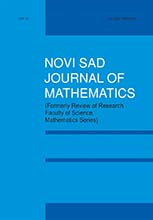eLibrary of Mathematical Instituteof the Serbian Academy of Sciences and Arts> Home / All Journals / Journal /Novi Sad Journal of MathematicsPublisher: Department of Mathematics and Informatics, Faculty of Sciences, Novi SadISSN: 1450-5444 (Print), 2406-2014 (Online)Issue: 44_2Date: 2014Journal Homepage

 Multipliers and convolutors in the space of tempered ultradistributions 1 - 18 Pavel Dimovski, Bojan Prangoski and Daniel Velinov
AbstractKeywords: tempered ultradistributions; multipliers; convolutorsMSC: 46F05
 $\Gamma$-nearrings with generalized $\Gamma$-derivations 19 - 28 Mohd Rais Khan and Mohammad Mueenul Hasnain
AbstractKeywords: $\Gamma$-nearring; prime $\Gamma$-nearring; $\Gamma$-derivation; generalized $\Gamma$-derivationMSC: 16Y30 16W25;16U80
 Generalized nonlinear variational inequalities 29 - 40 Balwant Singh Thakur and Suja Varghese
AbstractKeywords: generalized nonlinear variational inequality; fixed point problem; relaxed monotone operator; relaxed cocoercive mapping; nonexpansive mappingMSC: 47J20 65K10;65K15;90C33
 On $C$-Bochner curvature tensor of $(k,\mu )$-contact metric manifolds 41 - 51 Uday Chand De and Sujit Ghosh
AbstractKeywords: $C$-Bochner semi-symmetric manifold; $(k;\mu)$-contact metric manifold; Einstein manifold; $\eta$-Einstein manifold; Sasakian manifoldMSC: 53C15 53C25
 An asymptotic numerical method for singularly perturbed weakly coupled system of convection-diffusion type differential difference equations 53 - 68 V. Subburayan and N. Ramanujam
AbstractKeywords: weakly coupled system; convection-diffusion type equation; differential difference equation; negative shift; boundary value problem; Shishkin meshMSC: 34K10 34K26;34K28
 Random bipartite graphs 69 - 75 Boris Sobot
AbstractKeywords: random graphs; bipartite graphs; independent familiesMSC: 05C80 05C63;05C60
 On Lorentzian $\alpha$-Sasakian manifolds admitting a type of semi-symmetric metric connection 77 - 88 Ajit Barman
AbstractKeywords: Lorentzian $\alpha$-Sasakian manifold; semi-symmetric metric connection; locally $\phi$-symmetric; $\xi$-projectively flatMSC: 53C15; 53C25
 Additive decomposition schemes for polynomial functions over fields 89 - 105 Miguel Couceiro, Erkko Lehtonen and Tamás Waldhauser
AbstractKeywords: function of several variables; arity gap; polynomial function; partial derivativeMSC: 08A40 12E05
 On fully $\omega_1$-$p^{\o+n}$-projective Abelian $p$-groups 107 - 120 Peter Danchev
AbstractKeywords: countable groups; direct sums of cyclic groups; $p^{\omega+n}$-projective groups; $\o_1$-$p^{\omega+n}$-projective groups; fully $\omega_1$-$p^{\omega+n}$-projective groupsMSC: 20K10
 Applications of the thick distributional calculus 121 - 135 Ricardo Estrada and Yunyun Yang
AbstractKeywords: thick points; delta functions; distributions; generalized functionsMSC: 46F10
Article page: 12>>

 Remote Address: 18.206.13.203 • Server: elib.mi.sanu.ac.rsHTTP User Agent: CCBot/2.0 (https://commoncrawl.org/faq/)